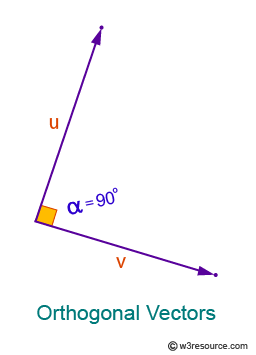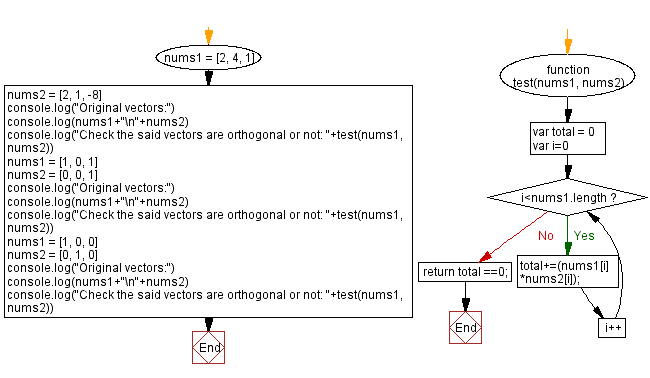# JavaScript: Check to given vectors are orthogonal or not

## JavaScript Math: Exercise-88 with Solution

Write a JavaScript program to check whether two vectors are orthogonal or not.

Two vectors are orthogonal if they are perpendicular to each other. i.e. the dot product of the two vectors is zero.
Let u and v are two vectors. The vectors u, v will be orthogonal if they are perpendicular, i.e., they form a right angle, or if the dot product they yield is zero.
u⊥v or u•v=0

Visualisation:Test Data:
([2, 4, 1], [2, 1, -8]) -> true
([1, 0, 1], [0, 0, 1]) -> false
([1, 0, 0], [0, 1, 0]) -> true

Sample Solution:

HTML Code:

``````<!DOCTYPE html>
<html>
<meta charset="utf-8">
<title>JavaScript program to check two given vectors are orthogonal or not</title>
<body>

</body>
</html>
```
```

JavaScript Code:

``````function test(nums1, nums2) {
var total = 0
for(var i=0;i<nums1.length;i++){
total+=(nums1[i] *nums2[i]);
}
}

nums1 = [2, 4, 1]
nums2 = [2, 1, -8]
console.log("Original vectors:")
console.log(nums1+"\n"+nums2)
console.log("Check the said vectors are orthogonal or not: "+test(nums1, nums2))
nums1 = [1, 0, 1]
nums2 = [0, 0, 1]
console.log("Original vectors:")
console.log(nums1+"\n"+nums2)
console.log("Check the said vectors are orthogonal or not: "+test(nums1, nums2))
nums1 = [1, 0, 0]
nums2 = [0, 1, 0]
console.log("Original vectors:")
console.log(nums1+"\n"+nums2)
console.log("Check the said vectors are orthogonal or not: "+test(nums1, nums2))

```
```

Sample Output:

```Original vectors:
2,4,1
2,1,-8
Check the said vectors are orthogonal or not: true
Original vectors:
1,0,1
0,0,1
Check the said vectors are orthogonal or not: false
Original vectors:
1,0,0
0,1,0
Check the said vectors are orthogonal or not: true
```

Flowchart:Live Demo:

See the Pen javascript-math-exercise-88 by w3resource (@w3resource) on CodePen.

Improve this sample solution and post your code through Disqus

What is the difficulty level of this exercise?

Test your Programming skills with w3resource's quiz.

﻿